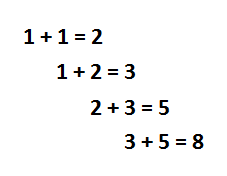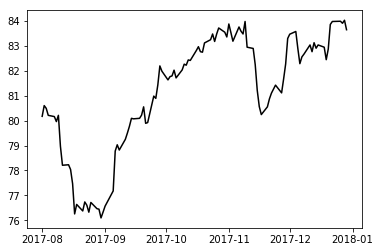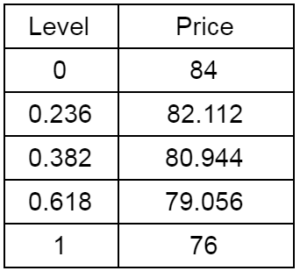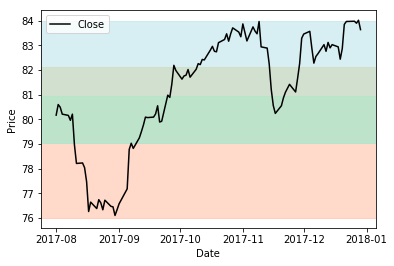# Fibonacci Retracement Trading Strategy In PythonFibonacci trading tools are used for determining support/resistance levels or to identify price targets. It is the presence of Fibonacci series in nature which attracted technical analysts’ attention to use Fibonacci for trading. Fibonacci numbers work like magic in finding key levels in any widely traded security. In this article, I will explain one of the famous Fibonacci trading strategy: retracement to identify support level.

### Fibonacci sequence

Fibonacci sequence is a series of numbers, starting with zero and one, in which each number is the sum of the previous two numbers.The Fibonacci sequence is 0, 1, 1, 2, 3, 5, 8, 13, 21, 34, 55, 89, 144, 233, 377, 610……
It extends to infinity and can be summarized using below formula:

Xn = Xn-1 + Xn-2

### What are some interesting facts about the Fibonacci sequence?

There are some interesting properties of the Fibonacci sequence. Divide any number in the sequence by the previous number; the ratio is always approximately 1.618.
```Xn/Xn-1 = 1.618
55/34 = 1.618
89/55 = 1.618
144/89 = 1.618```
1.618 is known as the golden ratio. I would suggest searching for the golden ratio examples on the Google images and you will be pleasantly astonished by the relevance of the ratio in nature.

Similarly, divide any number in the sequence by the next number; the ratio is always approximately 0.618.

```Xn/Xn+1 = 0.618
34/55 = 0.618
55/89 = 0.618
89/144 = 0.618```

0.618 expressed in percentage is 61.8%.
The square root of 0.618 is 0.786 (78.6%).

Similar consistency is found when any number in the sequence is divided by a number two places right to it.

```Xn/Xn+2 = 0.382
13/34 = 0.382
21/55 = 0.382
34/89 = 0.382```

0.382 expressed in percentage is 38.2%

Also, there is consistency when any number in the sequence is divided by a number three places right to it.

```Xn/Xn+3 = 0.236
21/89 = 0.236
34/144 = 0.236
55/233 = 0.236```

0.236 expressed in percentage terms is 23.6%.

The ratios 23.6%, 38.2%, 61.8%, and 78.6% are known as the Fibonacci ratios.

The Fibonacci ratios, 23.6%, 38.2%, and 61.8%, can be applied for time series analysis to find support level. Whenever the price moves substantially upwards or downwards, it usually tends to retrace back before it continues to move in the original direction. For example, if the stock price has moved from \$200 to \$250, then it is likely to retrace back to \$230 before it continues to move upward. The retracement level of \$230 is forecasted using the Fibonacci ratios.We can arrive at \$230 by using a simple math
Total up move = \$250 - \$200 = \$50
38.2% of up move = 38.2% * 50 = \$19.1
Retracement forecast = \$250 - \$19.1 = \$230.9

Any price level below \$230 provides a good opportunity for the traders to enter into new positions in the direction of the trend. Likewise, we can calculate for 23.6%, 61.8% and the other Fibonacci ratios.

### How to find Fibonacci retracement levels?

As we now know, retracements are the price movements that go against the original trend. To forecast the Fibonacci retracement level we should first identify the total up move or total down move. To mark the move, we need to pick the most recent high and low on the chart.

Let’s take an example of Exxon Mobil to understand the Fibonacci retracement construction

```# To import stock prices
from pandas_datareader import data as pdr
# To plot
import matplotlib.pyplot as plt
fig, ax = plt.subplots()
ax.plot(df.Close, color='black')```As seen from the graph, there is substantial upward movement in the price from September 2017 to end of October 2017. In this case, the minimum price is \$76 and maximum price is \$84. The \$8 is the total up move.

`price_min = 76 #df.Close.min()`
`price_max = 84 #df.Close.max()`

The retracement levels for Fibonacci ratios of 23.6%, 38.2% and 61.8% are calculated as follows:

```# Fibonacci Levels considering original trend as upward move
diff = price_max - price_min
level1 = price_max - 0.236 * diff
level2 = price_max - 0.382 * diff
level3 = price_max - 0.618 * diff

print "Level", "Price"
print "0 ", price_max
print "0.236", level1
print "0.382", level2
print "0.618", level3
print "1 ", price_min

ax.axhspan(level1, price_min, alpha=0.4, color='lightsalmon')
ax.axhspan(level2, level1, alpha=0.5, color='palegoldenrod')
ax.axhspan(level3, level2, alpha=0.5, color='palegreen')
ax.axhspan(price_max, level3, alpha=0.5, color='powderblue')

plt.ylabel("Price")
plt.xlabel("Date")
plt.legend(loc=2)
plt.show()```

Output:The first retracement level at 23.6% is \$82.10, the second retracement level at 38.6% is \$80.90, and the next retracement level at 61.8% is \$79.05. During mid-November, the Exxon Mobil stock price went down to \$80.40, (falling below 38.6% retracement level) and then continued the upward movement.

### How to use the Fibonacci retracement trading strategy?

The retracement levels can be used in a situation where you wanted to buy a particular stock but you have not been able to because of a sharp run-up in the stock price. In such a situation, wait for the price to correct to Fibonacci retracement levels such as 23.6%, 38.2%, and 61.8% and then buy the stock.The ratios 38.2% and 61.8% are the most important support levels.

This Fibonacci retracement trading strategy is more effective over a longer time interval and like any indicator, using the strategy with other technical indicators such as RSI, MACD, and candlestick patterns can improve the probability of success.

Good luck with Fibonacci trading :)

### Next Step

There are many sources for getting data from various cryptocurrencies available on the net. In our post 'Cryptocurrencies Trading Strategy With Data Extraction Technique' learn the use of python library coinmarketcap to fetch data. To learn more Quantitative trading strategies, you can go through the Quantitative Trading bundle.

Disclaimer: All investments and trading in the stock market involve risk. Any decisions to place trades in the financial markets, including trading in stock or options or other financial instruments is a personal decision that should only be made after thorough research, including a personal risk and financial assessment and the engagement of professional assistance to the extent you believe necessary. The trading strategies or related information mentioned in this article is for informational purposes only.